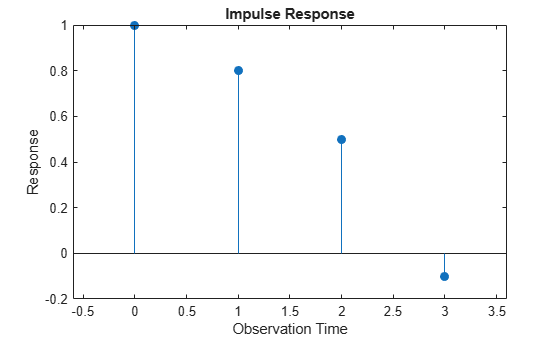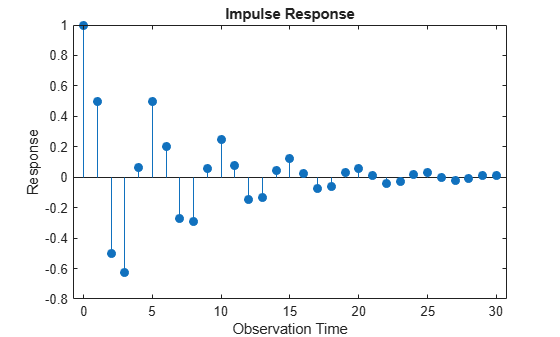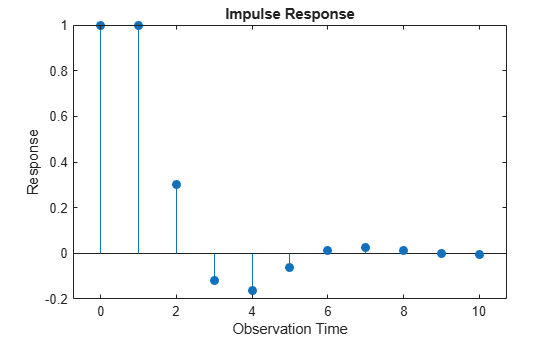## Plot the Impulse Response Function

### Moving Average Model

This example shows how to calculate and plot the impulse response function for a moving average (MA) model. The MA(q) model is given by

`${y}_{t}=\mu +\theta \left(L\right){\epsilon }_{t},$`

where $\theta \left(L\right)$ is a q-degree MA operator polynomial, $\left(1+{\theta }_{1}L+\dots +{\theta }_{q}{L}^{q}\right).$

The impulse response function for an MA model is the sequence of MA coefficients, $1,{\theta }_{1},\dots ,{\theta }_{q}.$

Create MA Model

Create a zero-mean MA(3) model with coefficients ${\theta }_{1}=0.8$, ${\theta }_{2}=0.5$, and ${\theta }_{3}=-0.1.$

`Mdl = arima('Constant',0,'MA',{0.8,0.5,-0.1});`

Step 2. Plot the impulse response function.

`impulse(Mdl)`For an MA model, the impulse response function cuts off after q periods. For this example, the last nonzero coefficient is at lag q = 3.

### Autoregressive Model

This example shows how to compute and plot the impulse response function for an autoregressive (AR) model. The AR(p) model is given by

`${y}_{t}=\mu +\varphi \left(L{\right)}^{-1}{\epsilon }_{t},$`

where $\varphi \left(L\right)$ is a $p$-degree AR operator polynomial, $\left(1-{\varphi }_{1}L-\dots -{\varphi }_{p}{L}^{p}\right)$.

An AR process is stationary provided that the AR operator polynomial is stable, meaning all its roots lie outside the unit circle. In this case, the infinite-degree inverse polynomial, $\psi \left(L\right)=\varphi \left(L{\right)}^{-1}$, has absolutely summable coefficients, and the impulse response function decays to zero.

Step 1. Specify the AR model.

Specify an AR(2) model with coefficients ${\varphi }_{1}=0.5$ and ${\varphi }_{2}=-0.75$.

`modelAR = arima('AR',{0.5,-0.75});`

Step 2. Plot the impulse response function.

Plot the impulse response function for 30 periods.

`impulse(modelAR,30)`The impulse function decays in a sinusoidal pattern.

### ARMA Model

This example shows how to plot the impulse response function for an autoregressive moving average (ARMA) model. The ARMA(p, q) model is given by

`${y}_{t}=\mu +\frac{\theta \left(L\right)}{\varphi \left(L\right)}{\epsilon }_{t},$`

where $\theta \left(L\right)$ is a q-degree MA operator polynomial, $\left(1+{\theta }_{1}L+\dots +{\theta }_{q}{L}^{q}\right)$, and $\varphi \left(L\right)$ is a p-degree AR operator polynomial, $\left(1-{\varphi }_{1}L-\dots -{\varphi }_{p}{L}^{p}\right)$.

An ARMA process is stationary provided that the AR operator polynomial is stable, meaning all its roots lie outside the unit circle. In this case, the infinite-degree inverse polynomial, $\psi \left(L\right)=\theta \left(L\right)/\varphi \left(L\right)$ , has absolutely summable coefficients, and the impulse response function decays to zero.

Specify ARMA Model

Specify an ARMA(2,1) model with coefficients ${\varphi }_{1}$ = 0.6, ${\varphi }_{2}=-0.3$, and ${\theta }_{1}=0.4$.

`Mdl = arima('AR',{0.6,-0.3},'MA',0.4);`

Plot Impulse Response Function

Plot the impulse response function for 10 periods.

`impulse(Mdl,10)`